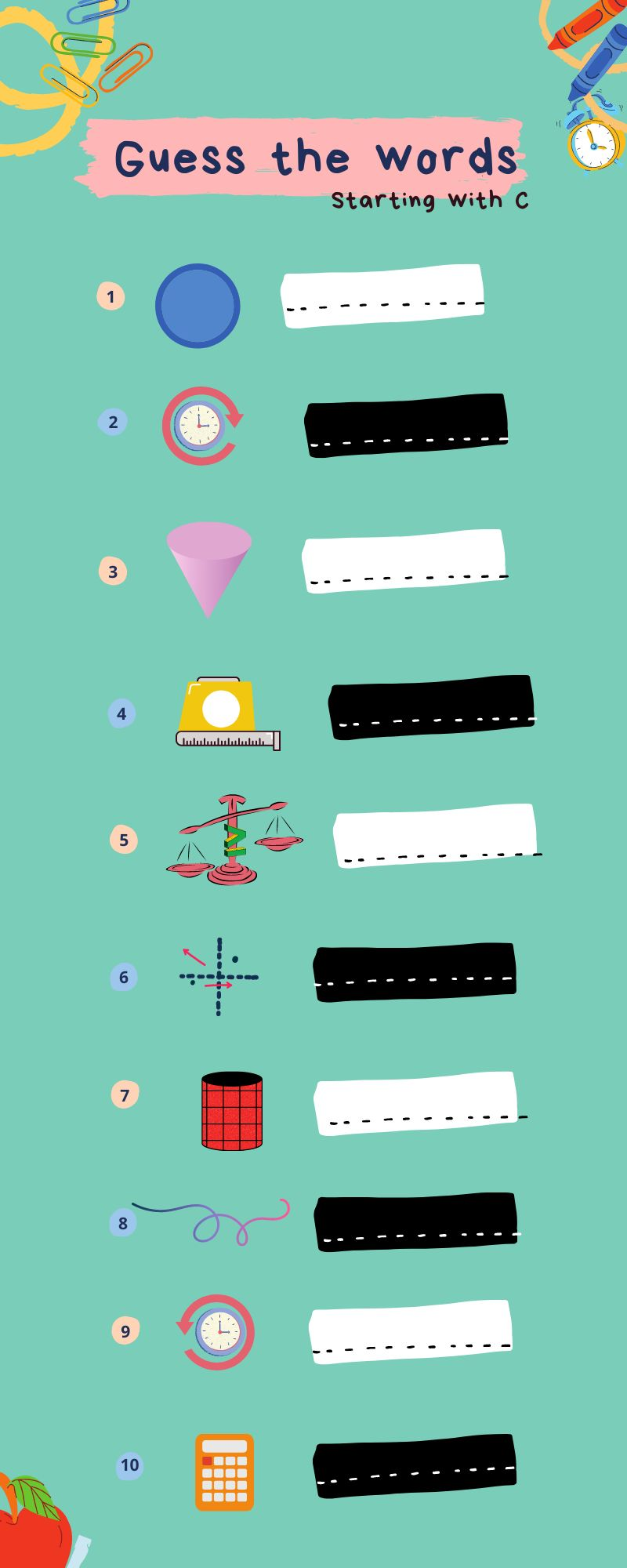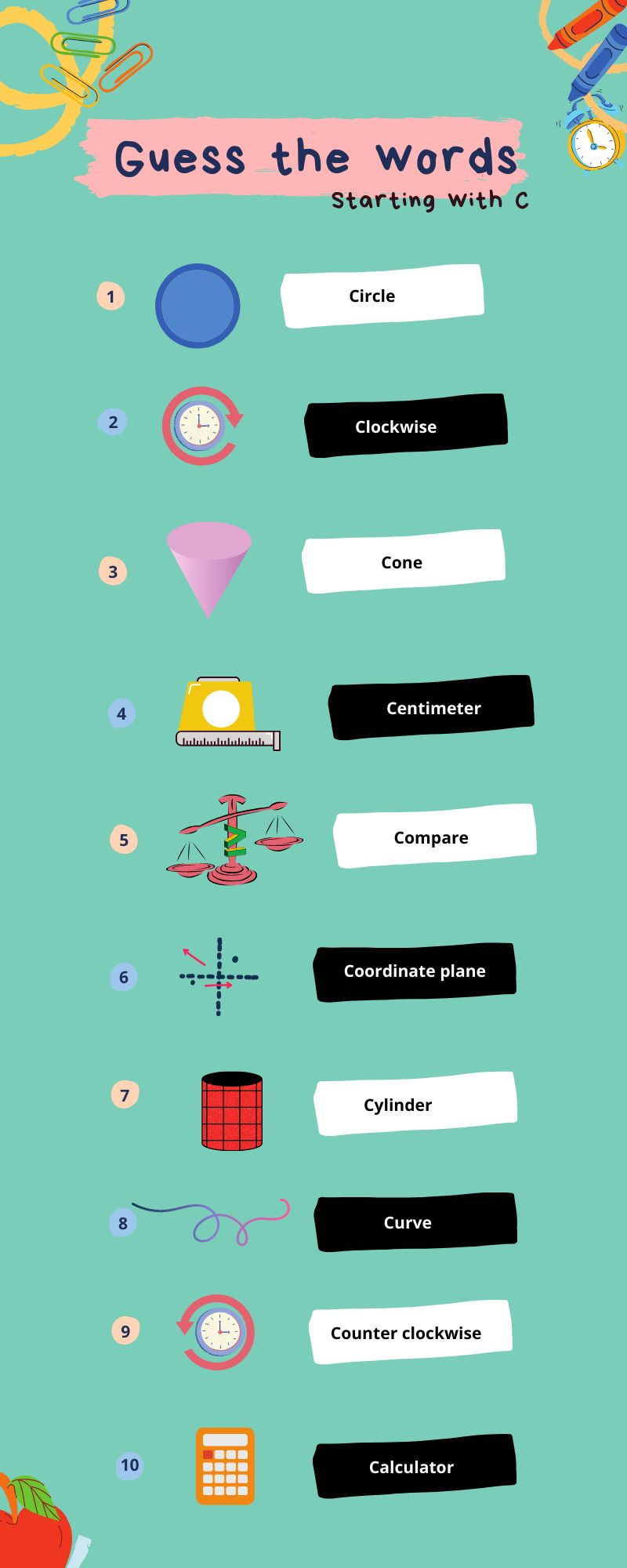Courses
Courses for Kids
Free study material
Free LIVE classes
More## List of Maths Words with Letter C

Maths is fascinating because it allows you to communicate a lot of information with just a few equations and graphs. Treat maths as a language, as you advance to more precise explanations and logical reasoning. Treating maths as a language completely removes the idea of challenging maths problems or difficult maths problems from your thoughts. There's nothing to be scared of in difficult maths problems; it's just that you haven't explored deeply enough mathematics to appreciate its simplicity and relatability. In this article, we are going to learn a few words which start with the letter see and also look at an activity to remember the words.

## Maths Words With C

Calculator: A device for doing arithmetical calculations.

Calendar: A calendar is a diagram that depicts the days of the week and their corresponding dates in the month.

Cancellation: If the numerator and denominator both have the same value, they cancel out.

Capacity: A container's capacity is the amount of liquid it can hold.

Capital: The money or other property invested by the partners in a company's capital.

Coordinate System: A conventional method of identifying locations in the plane that uses pairs of integers expressing distances along two fixed intersecting number lines, known as the axes. Both axes are perpendicular to one another and intersect at the origin. Rene Descartes, a French mathematician, is honoured with the name of the system. A rectangular coordinate system is another name for it.

Cartesian Coordinates: These are a set of numbers in the form of "an ordered pair" that is officially called "cartesian coordinates" ( x, y ).

Cartesian Plane: The Cartesian Plane is a plane with an x and y-axis.

Celsius: It is a temperature measurement scale seen on some thermometers.

Centre: The point inside a circle from which all other points on the circle are equally far is called the centre.

A centimetre: A centimeter is a metric measuring unit. One centimetre (cm) equals ten millimetres (mm); one metre equals 100 centimetres.

Central Angle: A central angle is an angle formed by two radii of a circle intersecting.

Centroid: The centroid of a figure is its centre mass. The intersection of a triangle's medians is its centroid.

Century: A century is a one-hundred-year period.

Chance: It is a technique of representing the chance of an event as a percentage.

Chord: It is a line segment that connects two points on the circumference of a circle.

Chronological Order: When dates or periods are presented from earliest to latest, they are in chronological order.

Coefficients: In a mathematical phrase, coefficients are the numbers in front of the letters.

Combinations: These are the various ways in which you can select items from a group of items.

Commission: It is a type of fee paid to an agent for completing a transaction.

The Common Denominator: Addition and subtraction of fractions require a common denominator, which is a multiple shared by the denominators of two or more fractions.

Common Year: A year having 365 days is referred to as a "common year,".

Commutative Property: This property of multiplication and addition indicates that you can alter the order of integers being added or multiplied without impacting the expression's value. A real-number condition that states that for any two real numbers a and b, a + b = b + a and a x b = b x a.

Compass: A compass is a tool for drawing circles and arcs.

Complementary Angles: These are two angles whose sum is 900.

Complex Fraction: a fraction with a fractional numerator, denominator, or both.

Complex Number: The sum of a real and an imaginary number is called a complex number.

Composite: A number with more than two factors is called a composite.

Composite Number: An integer with multiple integral factors except unity and itself is called a composite number.

Compound Ratio: The product of two or more simple ratios is called a compound ratio.

Compute: to answer difficulties that require the use of numbers.

Concave: Concave means curved from inside.

Concave Polygon: A concave polygon is one in which one or more interior angles are higher than 180 degrees.

Concave Up: When a curve is concave, it means it is curved like the inside of a bowl, with the two ends of the curve pointing up.

Concentric Circles: These are circles with the same centre but different radii.

Concrete Number: It is a number that refers to a specific unit.

Conditional Equation: A conditional equation is one in which the truth or falsity of the equation is determined by the values chosen to replace the variables.

Conditional Probability: It is defined as the likelihood of one event occurring if another event also occurs.

Classification of Data: The categorization of data for the most effective use is referred to as data classification. The process of arranging objects into homogeneous groups or classes based on shared characteristics is known as classification.

Concurrency in Straight Lines: When three or more straight lines intersect at the same point, the common point is referred to as the point of concurrency.

Circumcentre of the Triangle: The circumcentre of a triangle is the point at which the perpendicular bisector of its sides intersects. The circumcentre is denoted by S.

## Let’s Learn Words with Images

We have given some of the images with reference to the discussion above. Can you name these images? Try without looking at the answers that are provided below.Image Shows the Pictorial Representation of Mathematical Elements with Fill in the BlanksImage Shows the Pictorial Representation of Mathematical Elements Whose Names Starts with C

## Conclusion

If you approach mathematics properly, it can be fun. Maths is actually a game, one which sharpens your brain and improves your concentration. People today have a more positive and pleasant attitude toward mathematics than they did in the past, which makes it more interesting. Knowing that maths is a mindful activity rather than a task is the golden rule. We learned and understood some maths words that begin with the letter C in this article.

Last updated date: 24th Sep 2023
Total views: 119.7k
Views today: 1.19k

## FAQs on Let’s Learn Some Maths Words which Start with C

1. What is a Cylinder?

A geometrical shape with two parallel straight sides having two circular parts such that one is at the top and the other is at the bottom.

2. What is Compound Interest?

The interest also earns interest in compound interest. Money is said to be lent in this context when, at the end of a year or other fixed period, the interest that has become due is added to the sum, and the total amount becomes the principal for the next period. This procedure should be repeated until the amount for the previous period is found. The C.I. is the difference between the final amount and the original principal.

3. Explain the maths word ‘Cuboid’.

A cuboid is a solid shape with six rectangular faces. As a result, it resembles a box with rectangular sides on all sides. The square cuboid is a special case of the cuboid, with two (or more) opposite faces squares. The cube is a special case of the square cuboid, with all faces being squares. The square cuboid is also known as a right square prism.